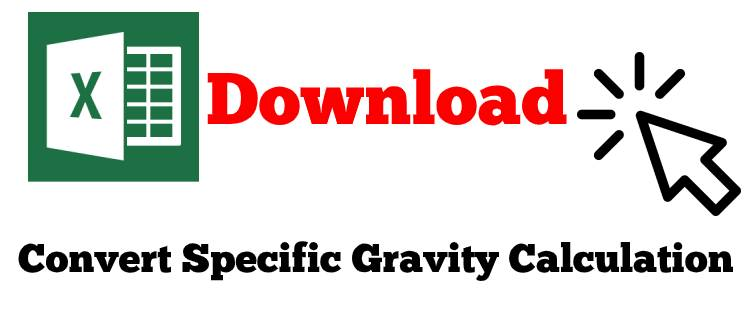# Convert specific gravity to mud weight (ppg and lb/ft3) and pressure gradient (psi/ft)

Referring to the previous article, Calculate Specific Gravity (SG) in oilfield unit , you get specific gravity (SG) from mud weight (ppg and lb/ft3) and pressure gradient (psi/ft) but you may need to reverse calculation from specific gravity (SG) to mud weight or pressure gradient (psi/ft). In this article, you will learn how to how to convert specific gravity to mud weight  and pressure gradient in both oilfield and metric unit by using simple formulas as follows:

## 1. Convert specific gravity to mud weight in pounds per gallon (ppg)

Mud weight in ppg = specific gravity (SG) × 8.33

Example: specific gravity = 1.50
mud wt, ppg = 1.50 × 8.33
mud wt = 12.5 ppg

## 2. Convert specific gravity to mud weight in lb/ft3

Mud weight in lb/ft3 = specific gravity × 62.4

Example: specific gravity = 1.50
Mud weight in lb/ft3 = 1.50 × 62.4 lb/ft3
Mud weight in lb/ft3 = 93.6 lb/ft3

## 3. Convert specific gravity to pressure gradient in psi/ft

Pressure gradient in psi/ft = specific gravity (SG) × 0.433

Example: specific gravity = 1.5
Pressure gradient in psi/ft  = 1.5 × 0.433
Pressure gradient in psi/ft = 0.650 psi/ft

## 4. Convert specific gravity to mud weight in kg/m3

Mud weight in kg/m3 = specific gravity × 1000

Example: specific gravity = 1.5

Mud weight in kg/m3 = 1.5 × 1,000

Mud weight in kg/m3 = 1,500

## 5. Convert specific gravity to mud weight in g/cc

Mud weight in g/cc = specific gravity × 1.0

Example: specific gravity = 1.5

Mud weight in  g/cc = 1.5 × 1.0

Mud weight in g/cc  = 1.5

## 6. Convert specific gravity to pressure gradient in kPa/m

Pressure gradient in kPa/m = specific gravity (SG) × 9.81

Example: specific gravity = 1.5
Pressure gradient in kPa/m  = 1.5 × 9.81
Pressure gradient in kPa/m = 14.7

Please also find the Excel sheet  for  this topic.Ref books:

Lapeyrouse, N.J., 2002. Formulas and calculations for drilling, production and workover, Boston: Gulf Professional publishing.

Bourgoyne, A.J.T., Chenevert , M.E. & Millheim, K.K., 1986. SPE Textbook Series, Volume 2: Applied Drilling Engineering, Society of Petroleum Engineers.

Mitchell, R.F., Miska, S. & Aadny, B.S., 2011. Fundamentals of drilling engineering, Richardson, TX: Society of Petroleum Engineers.

Share the joy